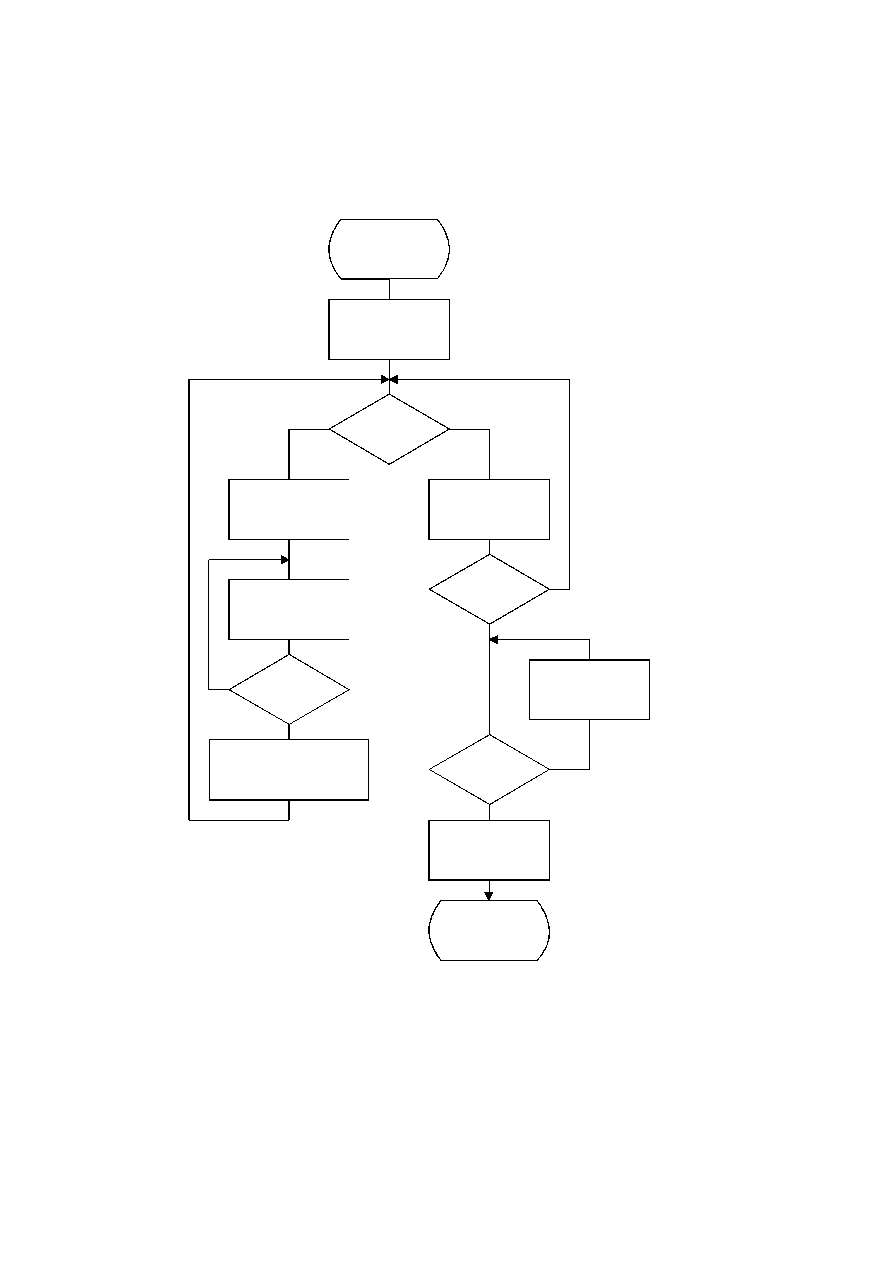ISO/IEC 10918-1 : 1993(E)
Figure K.3 gives the procedure for adjusting the BITS list so that no code is longer than 16 bits. Since symbols are paired
for the longest Huffman code, the symbols are removed from this length category two at a time. The prefix for the pair
(which is one bit shorter) is allocated to one of the pair; then (skipping the BITS entry for that prefix length) a code word
from the next shortest non-zero BITS entry is converted into a prefix for two code words one bit longer. After the BITS
list is reduced to a maximum code length of 16 bits, the last step removes the reserved code point from the code length
count.
TISO1750-93/d113
I = 32
Yes
No
J = I ­ 1
I = I ­ 1
J = J ­ 1
I = I ­ 1
Done
Yes
No
No
No
Yes
Yes
BITS(I) = BITS(I) ­ 1
BITS(I) = BITS(I) ­ 2
BITS(I ­ 1) = BITS(I ­ 1) + 1
BITS(J + 1) = BITS(J + 1) + 2
BITS(J) = BITS (J) ­ 1
BITS(I) > 0
?
BITS(J) > 0
?
BITS(I) = 0
?
I = 16
?
Figure K.3 ­ Procedure for limiting code lengths to 16 bits
Figure K.3 [D113] = 20 cm = 782 %
CCITT Rec. T.81 (1992 E)
147